xProjectile motionEncyclopedia
The motion in which a body is thrown or projected is called Projectile motion.
The path followed by a projectile is called its trajectory
Trajectory
A trajectory is the path that a moving object follows through space as a function of time. The object might be a projectile or a satellite, for example. It thus includes the meaning of orbit—the path of a planet, an asteroid or a comet as it travels around a central mass...

, which is directly influenced by gravity.

The initial velocity v0 can be written as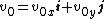The components v0x and v0y can be found if the angle θ0 is known:and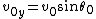The horizontal motion and the vertical motion are independent of each other ; that is, neither motion affects the other.

Since there is no acceleration in the horizontal direction, the horizontal component of the velocity remains unchanged throughout the motion.

The vertical motion is the motion of a particle in free fall. Equations for free fall apply. For example,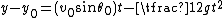.
Other useful equations for the vertical y-axis are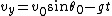,
and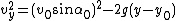.

Eliminating t between the following two equations,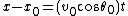and,
we obtain the equation of the path (the trajectory) of the projectile: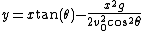Time to reach the maximum height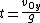Time to reach ground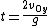Displacement in X direction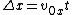Displacement in Y direction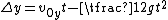Range of projectile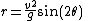Maximum height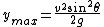## Parabolic trajectory

Since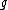,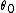, and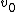are constants, the above equation is of the form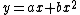,
in which a and b are constants. This is the equation of a parabola, so the path is parabolic.

The horizontal range R of the projectile is the horizontal distance the projectile has travelled when it returns to its initial height:

Note that R has its maximum value when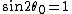, which necessarily corresponds to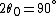or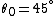.

• Kinematics
Kinematics
Kinematics is the branch of classical mechanics that describes the motion of bodies and systems without consideration of the forces that cause the motion....

• Range of a projectile
Range of a projectile
right|thumb|250 px|The path of this projectile launched from a height y0 has a range d.In physics, assuming a flat Earth with a uniform gravity field, a projectile launched with specific initial conditions will have a predictable range. As in Trajectory of a projectile, we will use:The following...

• Trajectory
Trajectory
A trajectory is the path that a moving object follows through space as a function of time. The object might be a projectile or a satellite, for example. It thus includes the meaning of orbit—the path of a planet, an asteroid or a comet as it travels around a central mass...

• Trajectory of a projectile
Trajectory of a projectile
In physics, the ballistic trajectory of a projectile is the path that a thrown or launched projectile will take under the action of gravity, neglecting all other forces, such as friction from air resistance, without propulsion....